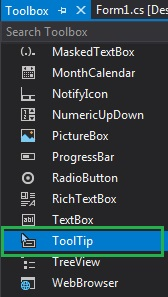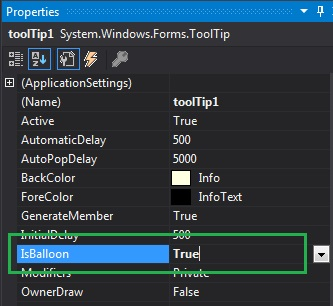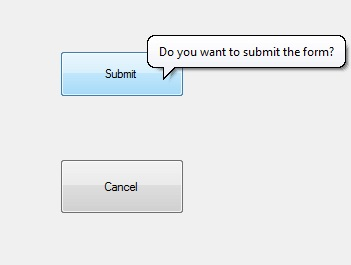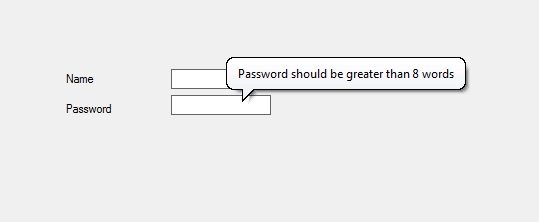# How to make a balloon ToolTip window in C#?

In Windows Forms, the ToolTip represents a tiny pop-up box which appears when you place your pointer or cursor on the control and the purpose of this control is it provides a brief description about the control present in the windows form. In ToolTip, you are allowed to change the shape of the ToolTip window by using IsBalloon Property.
With the help of this property, you can change the ToolTip window from the standard rectangular window to the balloon window. You can set this property in two different ways:

1. Design-Time: It is the easiest way to set the value of the IsBalloon property as shown in the following steps:

• Step 1: Create a windows form as shown in the below image:
Visual Studio -> File -> New -> Project -> WindowsFormApp• Step 2: Drag the ToolTip from the ToolBox and drop it on the form. When you drag and drop this ToolTip on the form it will automatically add to the properties(named as ToolTip on ToolTip1) of every controls present in the current windows from.• Step 3: After drag and drop you will go to the properties of the ToolTip and set the value of the IsBalloon property.Output:2. Run-Time: It is a little bit trickier than the above method. In this method, you can set the IsBalloon property of ToolTip programmatically with the help of given syntax:

`public bool IsBalloon { get; set; }`

Here, the value of this property is of System.Boolean type. If the value of this property is set to true, then the ToolTip window is of balloon window. And if the value of this property is set to be false, then the ToolTip window is of the standard rectangular window. The default value of this property is false.

The following steps show how to set the IsBalloon Property of the ToolTip dynamically:

• Step 1: Create a ToolTip using the ToolTip() constructor is provided by the ToolTip class.
```// Creating a ToolTip
ToolTip t = new ToolTip();
```
• Step 2: After creating Tooltip, set the IsBalloon property of the Tooltip provided by the ToolTip class.
```// Setting the IsBalloon property
t.IsBalloon = true;
```
• Step 3: And last add this ToolTip to the controls using SetToolTip() method. This method contains the control name and the text which you want to display in the ToolTip box.
` t.SetToolTip(box1, "Name should start with Capital letter");`

Example:

 `using` `System; ` `using` `System.Collections.Generic; ` `using` `System.ComponentModel; ` `using` `System.Data; ` `using` `System.Drawing; ` `using` `System.Linq; ` `using` `System.Text; ` `using` `System.Threading.Tasks; ` `using` `System.Windows.Forms; ` ` `  `namespace` `WindowsFormsApp34 { ` ` `  `public` `partial` `class` `Form1 : Form { ` ` `  `    ``public` `Form1() ` `    ``{ ` `        ``InitializeComponent(); ` `    ``} ` ` `  `    ``private` `void` `Form1_Load(``object` `sender, EventArgs e) ` `    ``{ ` ` `  `        ``// Creating and setting the  ` `        ``// properties of the Label ` `        ``Label l1 = ``new` `Label(); ` `        ``l1.Location = ``new` `Point(140, 122); ` `        ``l1.Text = ``"Name"``; ` ` `  `        ``// Adding this Label control to the form ` `        ``this``.Controls.Add(l1); ` ` `  `        ``// Creating and setting the  ` `        ``// properties of the TextBox ` `        ``TextBox box1 = ``new` `TextBox(); ` `        ``box1.Location = ``new` `Point(248, 119); ` `        ``box1.BorderStyle = BorderStyle.FixedSingle; ` ` `  `        ``// Adding this TextBox ` `        ``// control to the form ` `        ``this``.Controls.Add(box1); ` ` `  `        ``// Creating and setting  ` `        ``// the properties of Label ` `        ``Label l2 = ``new` `Label(); ` `        ``l2.Location = ``new` `Point(140, 152); ` `        ``l2.Text = ``"Password"``; ` ` `  `        ``// Adding this Label  ` `        ``// control to the form ` `        ``this``.Controls.Add(l2); ` ` `  `        ``// Creating and setting the ` `        ``// properties of the TextBox ` `        ``TextBox box2 = ``new` `TextBox(); ` `        ``box2.Location = ``new` `Point(248, 145); ` `        ``box2.BorderStyle = BorderStyle.FixedSingle; ` ` `  `        ``// Adding this TextBox  ` `        ``// control to the form ` `        ``this``.Controls.Add(box2); ` ` `  `        ``// Creating and setting the  ` `        ``// properties of the ToolTip ` `        ``ToolTip t = ``new` `ToolTip(); ` `        ``t.Active = ``true``; ` `        ``t.AutoPopDelay = 4000; ` `        ``t.InitialDelay = 600; ` `        ``t.IsBalloon = ``true``; ` `        ``t.SetToolTip(box1, ``"Name should start with Capital letter"``); ` `        ``t.SetToolTip(box2, ``"Password should be greater than 8 words"``); ` `    ``} ` `} ` `} `

Output:My Personal Notes arrow_drop_upCheck out this Author's contributed articles.

If you like GeeksforGeeks and would like to contribute, you can also write an article using contribute.geeksforgeeks.org or mail your article to contribute@geeksforgeeks.org. See your article appearing on the GeeksforGeeks main page and help other Geeks.

Please Improve this article if you find anything incorrect by clicking on the "Improve Article" button below.

Article Tags :

Be the First to upvote.

Please write to us at contribute@geeksforgeeks.org to report any issue with the above content.